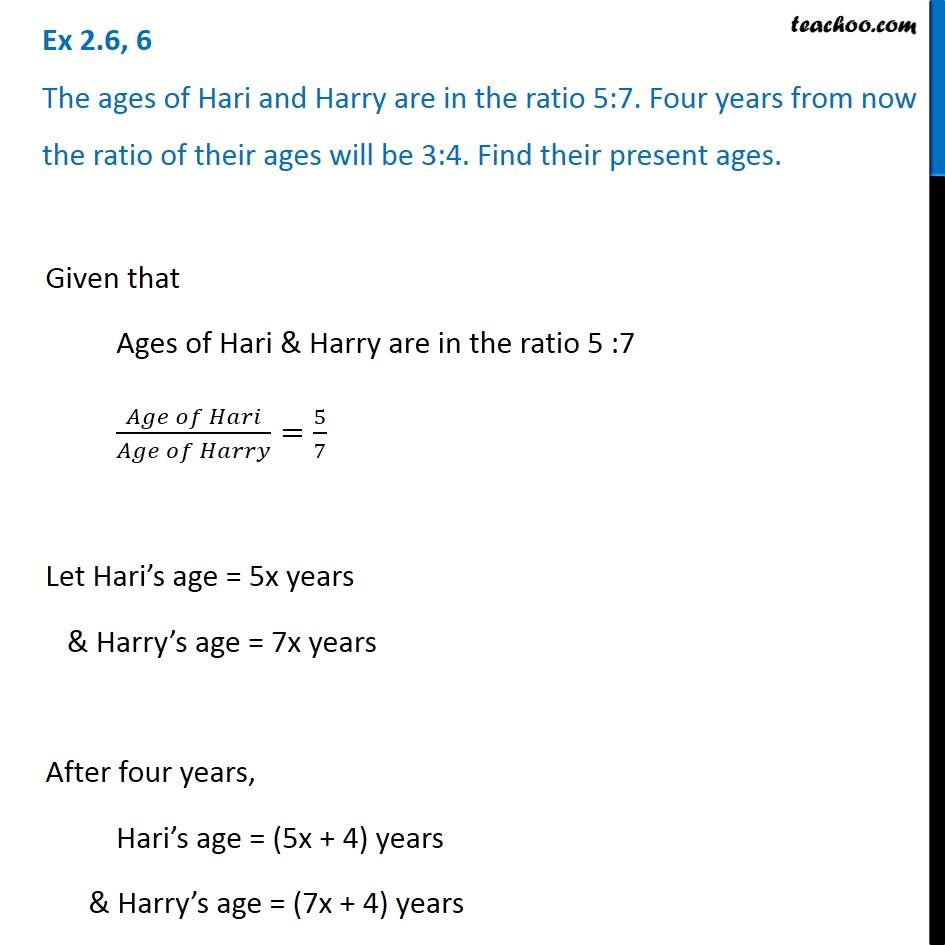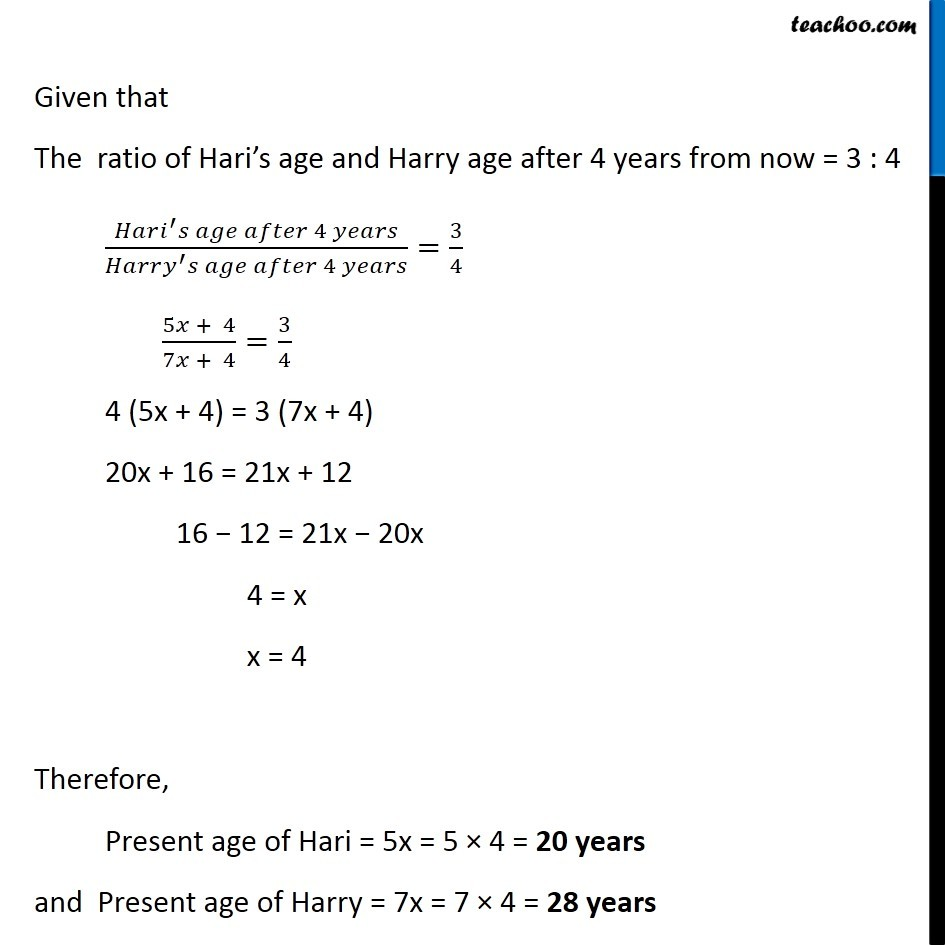Solving difficult equations - Word Problems

Chapter 2 Class 8 Linear Equations in One Variable
Serial order wiseLearn in your speed, with individual attention - Teachoo Maths 1-on-1 Class

### Transcript

Question 6 The ages of Hari and Harry are in the ratio 5:7. Four years from now the ratio of their ages will be 3:4. Find their present ages.Given that Ages of Hari & Harry are in the ratio 5 :7 ( )/( )=5/7 Let Hari s age = 5x years & Harry s age = 7x years After four years, Hari s age = (5x + 4) years & Harry s age = (7x + 4) years Given that The ratio of Hari s age and Harry age after 4 years from now = 3 : 4 ( ^ 4 )/( ^ 4 )=3/4 (5 + 4)/(7 + 4)=3/4 4 (5x + 4) = 3 (7x + 4) 20x + 16 = 21x + 12 16 12 = 21x 20x 4 = x x = 4 Therefore, Present age of Hari = 5x = 5 4 = 20 years and Present age of Harry = 7x = 7 4 = 28 years